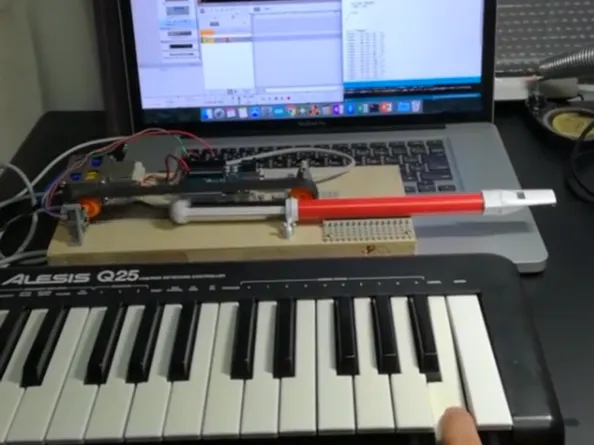Project tutorialMIDI Slide Whistle "MEMIDION"

I tried to make slide whistle move automatically by MIDI signal. It is MEMIDION.

• 1,995 views
• 9 respects

Apps and online services

I tried to make slide whistle move automatically by MIDI signal. It is MEMIDION.

MEMIDION can be operated as a musical instrument by MIDI keyboard, and it can be used as a MIDI sound source by moving with DAW. But no sound unless you breathe in....

Control was done by Arduino which made it into a USB-MIDI device.

MEMIDION Details

Motor (Caterpillar) control via motor driver DRV 8835 at Arduino.

Measure the distance of the tip of the slider of the whistle with the distance sensor VL6180X to control the slide amount.

Arduino USB-MIDI device conversion

Write Moco for LUFA firmware to Arduino and let Arduino recognize it as a MIDI device.

the detail is right below.

https://github.com/kuwatay/mocolufa

Setting of DAW software Reason

Anything is OK if DAW can output MIDI, but we will use Reason this time.

Place the external MIDI instrument and select "MocoLUFA" for the device.

Arduino IDE Code

Move the slider of the whistle with the MIDI signal from the MIDI keyboard or DAW and change the scale. The slide amount is controlled by the measurement value of distance sensor VL6180X.

I used the following as a MIDI library for Arduino.

https://github.com/FortySevenEffects/arduino_midi_library/

The library for distance sensor VL6180X is below.

https://github.com/pololu/vl6180x-arduino

#include <MIDI.h>
MIDI_CREATE_DEFAULT_INSTANCE();
#include <Wire.h>
#include <VL6180X.h>
uint8_t Note, NoteOld;
VL6180X sensor;
int meas;
int aim = 50;
int diff = 5;
int moveTime = 100;
void setup()
{
Wire.begin();
MIDI.begin();
pinMode(2, OUTPUT);
pinMode(3, OUTPUT);
sensor.init();
sensor.configureDefault();
sensor.setTimeout(500);
}
void loop()
{
//MIDI detection
switch(MIDI.getType()) {
case midi::NoteOn:
digitalWrite(2, HIGH);
digitalWrite(3, LOW);
delay(10);
digitalWrite(2, LOW);
digitalWrite(3, LOW);
Note = MIDI.getData1();    // Note = 48-72 ALESIS Q25
break;
case midi::NoteOff:
Note = 255;
break;
}
}
//Slide distance setting
if(Note == 57) aim = 22;  //A
if(Note == 58) aim = 33;  //A#
if(Note == 59) aim = 41;  //B
if(Note == 60) aim = 52;  //C
if(Note == 61) aim = 62;  //C#
if(Note == 62) aim = 71;  //D
if(Note == 63) aim = 81;  //D#
if(Note == 64) aim = 90;  //E
if(Note == 65) aim = 97; //F
if(Note == 66) aim = 103; //F#
if(Note == 67) aim = 109; //G
if(Note == 68) aim = 116; //G#
if(Note == 69) aim = 121; //A
if(Note == 70) aim = 126; //A#
if(Note == 71) aim = 129; //B
if(Note == 72) aim = 133; //C
//Distance sensor measurement
//Motor operation
if(meas > (aim - diff) && meas <  (aim + diff)){
digitalWrite(2, LOW);
digitalWrite(3, LOW);
}else if(meas > aim){
digitalWrite(2, LOW);
digitalWrite(3, HIGH);
}else if(meas < aim){
digitalWrite(2, HIGH);
digitalWrite(3, LOW);
}
}

Operation

The amount of slide will change with the MIDI keyboard.

Automatic performance as MIDI sound source with DAW is also possible. My breath has to keep blowing....

MIDI Slide Whistle "MEMIDION" Next Stage

Project tutorial by HomeMadeGarbage

• 2,741 views
• 15 respects

Minimal MIDI Drum Kit with 3D Printer

Project tutorial by ryokosaka

• 14,415 views
• 1 comment
• 38 respects

USB-BLE Wireless MIDI Adapters

Project tutorial by Joe Bowbeer

• 4,670 views
• 8 respects

Turn an Arduino Uno into a MIDI Controller: Guitar Pedals

Project tutorial by Johan van Vugt

• 5,561 views
• 1 comment
• 11 respects

Arduino-Based Pressure Cooker Whistle Indicator

Project tutorial by sourav344

• 1,735 views